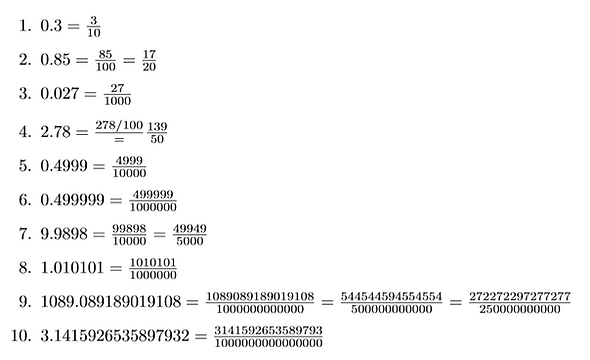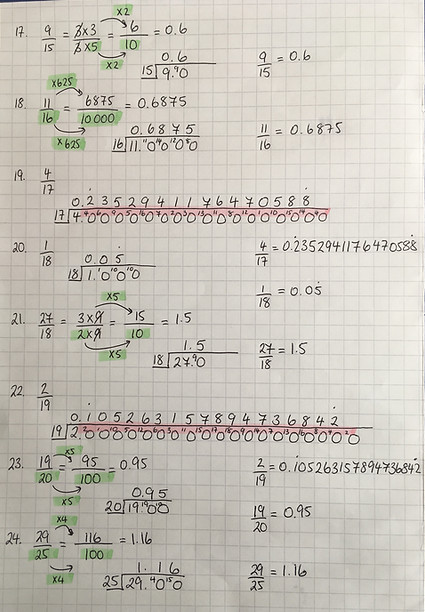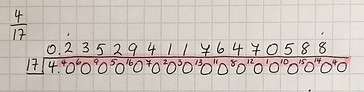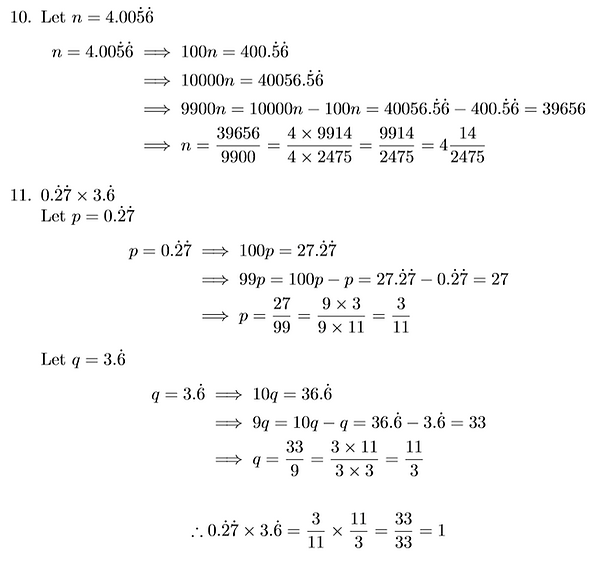top of page## Terminators## The General Terminator## Back to Bus StopsTerminating Decimals

The fractions in questions 1, 2, 4, 5, 7, 9, 11, 17, 18, 21, 23, 24, 26, 27, 29, 30 & 31 all have decimal expansions which terminate. These are also the questions in which we have an alternative method available to us other than classic 'bus stop' division. As the green highlight shows, in each of these cases we are able to use an integer multiplier to reach an equivalent fraction whose denominator is some power of 10. Why do we need the denominator to be a power of 10 in order to convert to decimals? Well because that's what decimals are, they chop up the value of a number so that it can be represented as the sum of multiples of each power of 10; how many tenths, how many hundredths, how many thousandths so on and so forth. This is the same mathematics that earlier allowed us to write any terminating decimal as a fraction just working in reverse. A terminating decimal can be written as a fraction because the last power of 10 we reach gives us the denominator, so if a fraction can be written with a denominator that is a power of 10 then it can be written as a terminating decimal.

Why though is it only these 14 of the 32 fractions which can have such a denominator? Have another look at the denominators of all the fractions that you have found give terminating decimals. You should see that the answer lies in prime factor decomposition. Any power of 10 has only the prime factors that 10 has: 2 and 5. So to reach a power of 10 denominator using equivalent fractions, we can start with a denominator that has any combination of 2s and 5s in its prime factor decomposition, but if there are any other primes then it becomes impossible (unless the intruding prime factors can be cancelled with the same factors in the numerator, as is the case in fractions 7, 17, 21, 26, 30 & 31). If an equivalent fraction with a power of 10 denominator can't be reached, then the decimal doesn't have anywhere to stop and so it doesn't. The denominators of all the fractions that give terminating decimals, when those fractions are written in simplest form, are made up only of 2s and 5s. The multipliers, also highlighted in green, are consequently also made up of only 2s and 5s, those needed to match up with every 5 and 2 in the denominator to form that all important power of 10.

Recurring Decimals

The fractions in questions 3, 6, 8, 10, 12, 13, 14, 15, 16, 19, 20, 22, 25, 28 & 32 therefore lead to decimal expansions which do not terminate. Instead the decimal expansions for these fractions at some point begin to repeat in a pattern, we call this recurring (note the lack of o in this word) because the same pattern repeats over and over forever. These are the fractions whose denominators cannot reach a power of 10 through integer multiplication, because they have prime factors other than 2 and 5, and so these are the fractions who do not properly 'fit' into a system which is built on powers of 10, the decimal system.

We already know that these fractions cannot give terminating decimals on account of the prime factorisation of their denominators, that doesn't explain though why they begin to repeat or whether all such fractions will lead to repetition.

While performing these conversions we understand that this repeating happens once we reach a remainder in our division that we've already encountered. For example, when dividing 2 by 3, we first get 0 remainder 2, so we must divide 20 by 3, which gives 6 with a remainder of 2 again, so again we must divide 20 by 3 which must lead to the same 6 with the same remainder of 2 so on and so forth. Hence we know the 6s will repeat forever.

The question that remains is whether any fraction with primes other than 2 and 5 in the composition of the denominator will give a decimal that at some point begins to repeat or are there cases where the decimal behaves differently? To investigate this, it is useful to look at fractions such as those in questions 8, 19 and 22. Look closely at the denominator in each of those fractions as well as the remainders reached in the division process, they are highlighted in pink.

When this fraction is written in simplest form, the denominator is 17. The decimal expansion is a repeating pattern 16 digits in length and in fact, it couldn't be any longer. You should notice that by the time we get to the 16th decimal place, we have met every number from 1 to 16 as a remainder in the division. We have met each remainder only once, if we hadn't then the pattern would have begun to repeat earlier. When we perform our next division we know we will have a remainder because the decimal doesn't terminate but we have already used all of the possible non-zero remainders for dividing by 17, they are exactly the integers from 1 to 16. If we went up to a remainder of 17 that wouldn't be a remainder at all as we would fit in another 17 exactly, a remainder of 18 then is another multiple of 17 fit in exactly with a remainder of 1, a remainder of 19 is a remainder of 2, so on and so forth. Dividing by 17 therefore limits the possible non-zero remainders, they can only be 1 through to 16. So, once we have 16 decimal places we must have met all 16 remainders, the 17th remainder has no choice but to be a repeat of one of those 16 and therefore the 17th decimal place is a repeat too. Once one repeat occurs, the cycle is locked in by the pattern of the remainders and so the pattern repeats forever.

This property is not unique to 17. When dividing by 101 there are only 100 possible non-zero remainders, so the decimal expansion of any fraction with a denominator of 101 must terminate or begin to repeat in 100 places or fewer. When dividing by n+1 there are only n possible non-zero remainders, so the decimal expansion of any fraction with a denominator of n+1 must terminate or begin to repeat in n places or fewer. Find any wild denominator you like, huge and prime as they come, still all of the fractions you can possibly make with it will all be bound to terminate or repeat at some point, it cannot be escaped.

For fractions into decimals then they are the only options, terminate or recur. But could decimals not behave in another way?## Forever to Playbottom of page